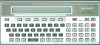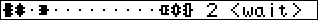##### Sharp PC-1500 : TRIO game

"Trio" for Sharp PC-1500 +4,8,16 KB or PC-1500A.
Adapted from an original program for Casio FX-702P published in "L'Ordinateur de Poche" #12, April 1983.
© Alain Ginsbach, L'Ordinateur de Poche, 1983; E. Beaurepaire, 2000.(*)
BASIC program - 2017 bytes.Game: an interesting little strategy game. The two players (you and the PC-1500) have three different pieces and play in successive turns. You must bring one of your pieces to the other end of the "board", or take all your opponent's pieces. One piece can take an ennemy by moving to the same location, but can not take its counterpart (symmetric piece). The small pieces can move one step forward or 3 steps back, the medium ones move 2 steps forward or 2 steps back, and the large ones move three steps forward or one step back. Game is won when one of the pieces has reached the other side, or when the opponent can't play its turn.

Running the program:
Type RUN or DEF A
Keys:
1/2/3 : select piece
+/- : select move
SPACE : give up

(*) This software is made available for documentation purpose only, since the PC-1500 is now an obsolete computer. This is not a commercial site. If you own a copyright on this program and do not want it to be available from this site, please inform me and I will remove it.

BASIC program.

1 "TRIO"REM PC-1500+4Ko or PC-1500A
10 "A"CLEAR :RANDOM :DIM A(22)
20 A\$="000008000000"
30 B\$="2A3E3E3E0800",C\$="143E7F3E1400",D\$="087F7F7F2A00"
40 E\$="083E223E2A00",F\$="143E633E1400",G\$="2A7F417F0800"
50 WAIT 0:CLS :PRINT " (1) TRIO (2) Rules"
55 ON VAL INKEY\$ +1GOTO 55,60,800
60 A(17)=3,A(18)=2,A(19)=1,A(20)=14,A(21)=15,A(22)=16
70 PRINT "Do you play (1)st/(2)nd ?"
75 M=VAL INKEY\$:IF M<>1AND M<>2GOTO 75
77 CLS
80 A(R+G)=Y,J=1-J,S=16+3*J,R=35-S,Q=S-16
90 FOR I=1TO 16:A(I)=0:NEXT I
100 FOR I=17TO 22
110 IF A(I)<>0LET A(A(I))=I-16
120 NEXT I
130 K\$="000000000000":GOTO 150
140 K\$="020151090600"
150 O=0
160 CURSOR 0:BEEP 1,50,30:FOR I=1TO 16:GPRINT @\$(A(I)+1);:NEXT I:PRINT 2-J
170 CURSOR 18:GPRINT K\$
180 IF Y=16-15*JGOTO 670
185 IF A(R+1)+A(R+2)+A(R+3)=0GOTO 670
190 CURSOR 19:IF J=M-1PRINT "<wait>":GOSUB 380:GOTO 270
195 PRINT " "
200 K\$=INKEY\$ :IF K\$=""GOTO 200
210 IF O=1GOTO 260
220 IF K\$=" "GOTO 700
230 FOR G=1TO 3
240 IF K\$=STR\$ GIF @\$(G+4-3*J)<>A\$LET O=1,K\$=@\$(G+4-3*J):BEEP 1,8,50:GOTO 170
250 NEXT G:GOTO 140
260 L=1:IF K\$<>"+"LET L=-1:IF K\$<>"-"GOTO 140
270 GOSUB 300:IF E=0GOTO 140
280 IF E=2LET @\$(Q+I+1)=A\$,A(S+I)=0
290 GOTO 80
300 X=A(R+G),T=G*L:IF T<0LET T=-T-4
310 IF J=0LET T=-T
320 Y=X+T:IF Y>0AND Y<17LET N=A(Y):IF N<>Q+GGOTO 340
330 E=0:RETURN
340 IF N=0LET E=1:RETURN
350 FOR I=1TO 3
360 IF N=Q+ILET E=2:RETURN
370 NEXT I:GOTO 330
380 P=0,B=(A(S+1)<>0)+(A(S-2)<>0)+(A(S+3)<>0)
390 FOR G=1TO 3
400 IF A(R+G)=0GOTO 640
410 FOR L=-1TO 1STEP 2
420 GOSUB 300:IF E=0GOTO 630
430 IF E=1LET E=0
440 IF E=2IF I<>1LET E=4
450 IF Y=15*J+1RETURN
455 IF E=2IF B=1RETURN
460 D=0,F=0,H=0,U=0
470 FOR I=-3TO 3:IF I=0GOTO 570
480 IF ABS I=GGOTO 570
490 Z=A(S+ABS I):IF Z=0GOTO 570
500 O=SGN (J-.5),C=O*(2*(1-SGN I)-ABS I)
510 IF X-Z=CLET D=2
520 IF X+T-Z=CLET F=2
530 Z=Z-O*I:IF X-Z=CLET H=1
540 IF X+T-Z=CLET U=1
550 Z=Z+O*4:IF X-Z=CLET H=1
560 IF X+T-Z=CLET U=1
570 NEXT I
580 W=(1+SGN (G-1))*(D-F)+E+(H-U+L)/2
590 IF G*L=-3LET N=(X-1)/3:IF N<>INT NLET W=W+1
600 IF P<>0IF W<VGOTO 630
610 IF P<>0IF W<>VIF RND 2=1GOTO 630
620 P=G*L,V=W
630 NEXT L
640 NEXT G
650 IF P=0GOTO 690
660 G=ABS P,L=SGN P:RETURN
670 IF J=M-1GOTO 690
680 CURSOR 16:PRINT " I WIN! ";:GOTO 710
690 CURSOR 16:PRINT " YOU WIN!";:GOTO 710
700 CURSOR 16:PRINT " GIVE UP";
710 BEEP 5,150,20:WAIT :PRINT ""
720 END
800 WAIT 120
810 PRINT "Goal:Reach the other side."
820 PRINT "A piece can take others..."
830 PRINT "...except its counterpart."
840 PRINT "1,2,3 : select piece"
850 PRINT "+,- : select move"
860 PRINT "SPACE : give up"
870 GOTO 50